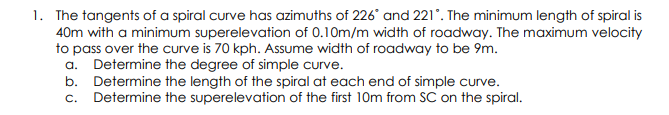# Question 1. The tangents of a spiral curve has azimuths of 226 and 221°. The minimum length of spiral is 40m with a minimum superelevation of 0.10m/m width of roadway. The maximum velocity to pass over the curve is 70 kph. Assume width of roadway to be 9m. a. Determine the degree of simple curve. b. Determine the length of the spiral at each end of simple curve. c. Determine the superelevation of the first 10m from SC on the spiral.QS0RRX The Asker · Civil EngineeringTranscribed Image Text: 1. The tangents of a spiral curve has azimuths of 226 and 221°. The minimum length of spiral is 40m with a minimum superelevation of 0.10m/m width of roadway. The maximum velocity to pass over the curve is 70 kph. Assume width of roadway to be 9m. a. Determine the degree of simple curve. b. Determine the length of the spiral at each end of simple curve. c. Determine the superelevation of the first 10m from SC on the spiral.
More
Transcribed Image Text: 1. The tangents of a spiral curve has azimuths of 226 and 221°. The minimum length of spiral is 40m with a minimum superelevation of 0.10m/m width of roadway. The maximum velocity to pass over the curve is 70 kph. Assume width of roadway to be 9m. a. Determine the degree of simple curve. b. Determine the length of the spiral at each end of simple curve. c. Determine the superelevation of the first 10m from SC on the spiral.